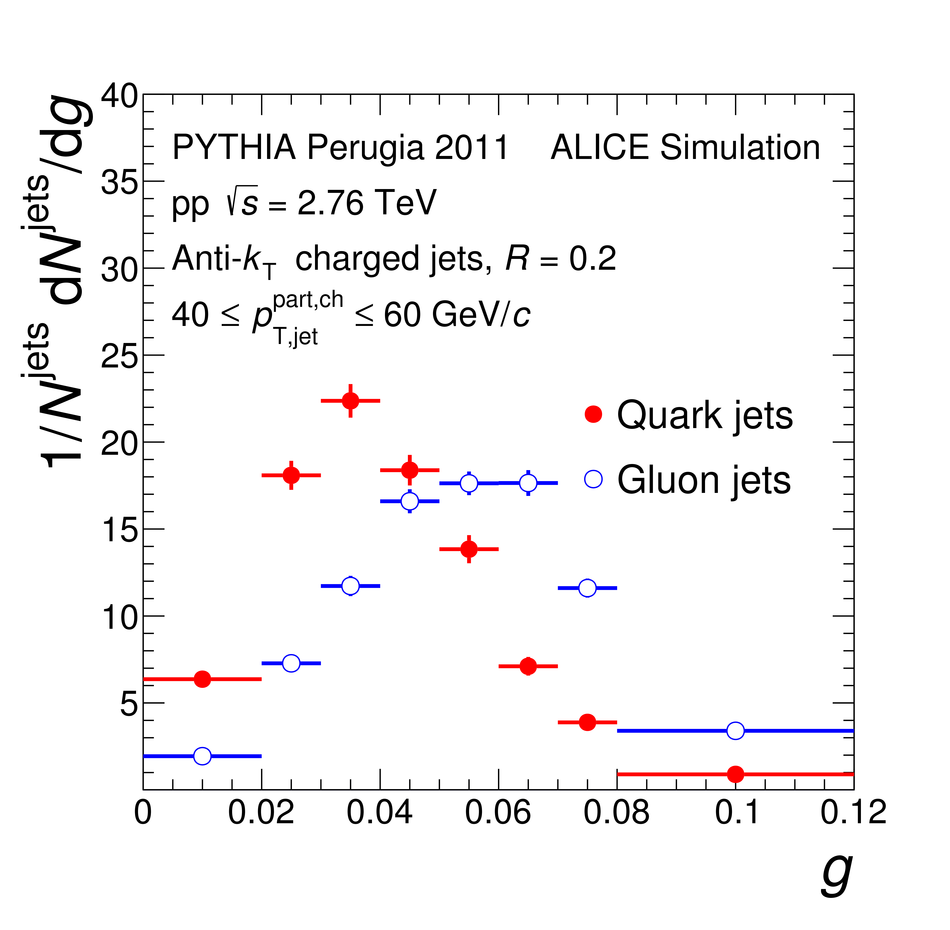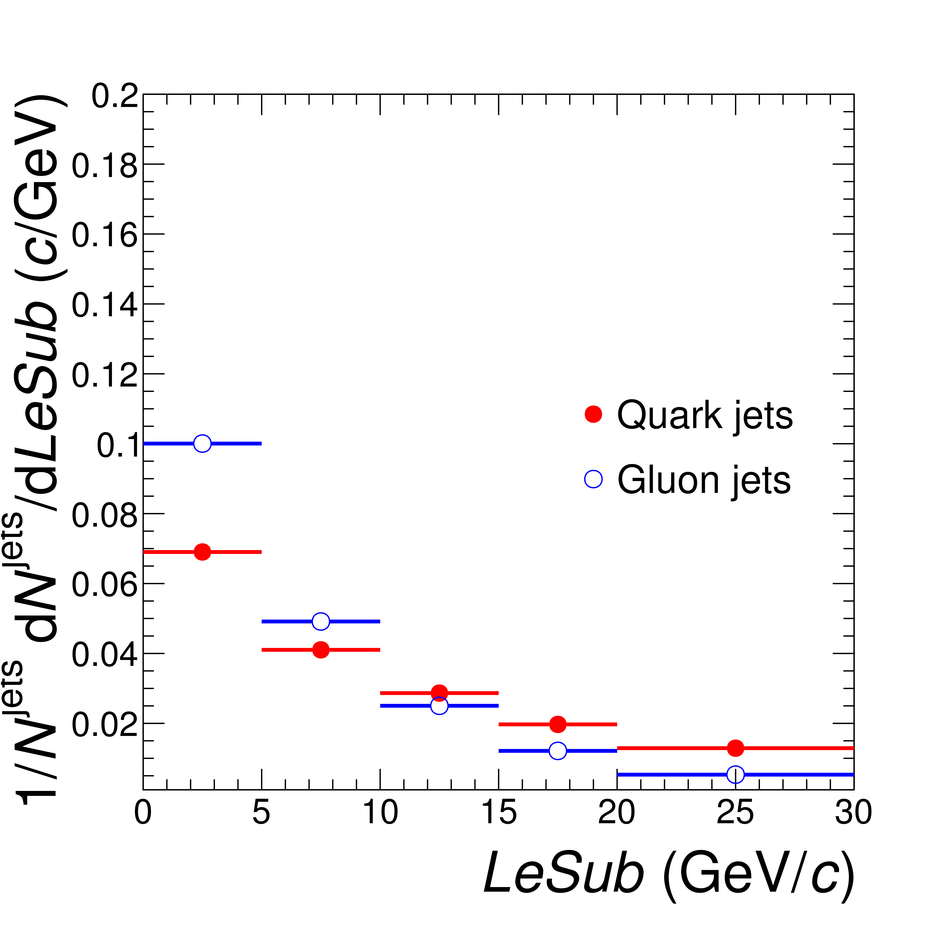# Figure 1

 $g$, $p_{\rm T}D$, and $LeSub$ for quark and gluon jets as obtained from PYTHIA Perugia 2011 simulations of pp collisions at $\sqrt{s}=2.76$\,TeV in the transverse momentum interval $40 \leq p_{\mathrm{T,jet}}^{\rm{part,ch}} \leq 60$\,GeV/$c$.